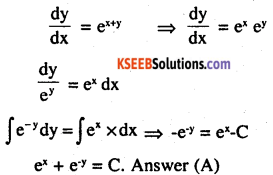# 2nd PUC Maths Question Bank Chapter 9 Differential Equations Ex 9.4

Students can Download Maths Chapter 9 Differential Equations Ex 9.4 Questions and Answers, Notes Pdf, 2nd PUC Maths Question Bank with Answers helps you to revise the complete Karnataka State Board Syllabus and score more marks in your examinations.

## Karnataka 2nd PUC Maths Question Bank Chapter 9 Differential Equations Ex 9.4

### 2nd PUC Maths Differential Equations NCERT Text Book Questions and Answers Ex 9.4

For each of the differential equations in Exercise 1 to 10, find the general solution:

Question 1.
$$\frac{d y}{d x}=\frac{1-\cos x}{1+\cos x}$$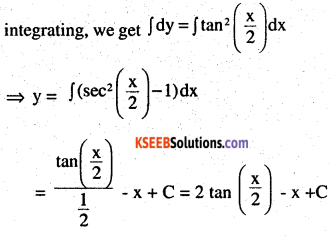which is required solutions of given differencial equation.Question 2.
$$\frac{d y}{d x}=\sqrt{4-y^{2}}(-2<y<2)$$Question 3.
$$\frac{d y}{d x}+y=1(y \neq 1)$$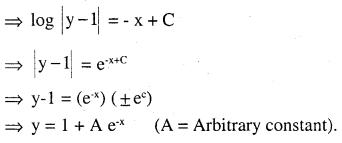Question  4.
sec x tan y dx + sec2 y tan x dy = 0Question 5.
(ex + ex) dy – (ex – ex) dx = 0Question 6.
$$\frac{d y}{d x}=\left(1+x^{2}\right)\left(1+y^{2}\right)$$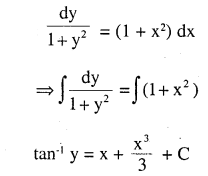Question 7.
y log y dx – x dy = 0Question 8.
$$x^{5} \times \frac{d y}{d x}$$Question 9.
$$\frac{d y}{d x}=\sin ^{-1} x$$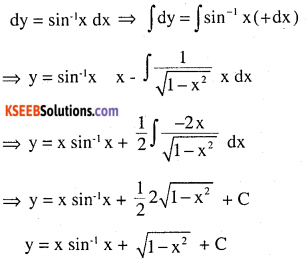Question 10.
ex tan y dx + (1 – ex) sec2 y dy = 0For each of the differential equations in Exercises 11 to 14, find a particular solution satisfying the given condition:

Question 11.
(x3 + x2 + x +1) $$\frac{\mathrm{d} y}{\mathrm{d} x}$$= 2x2 + x; y = 1
when x = o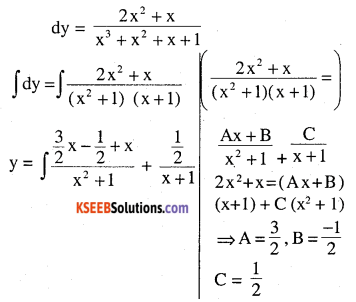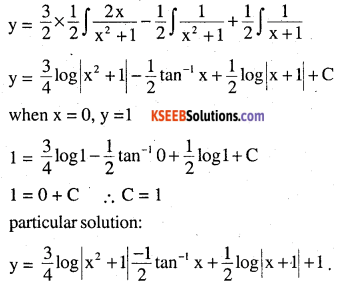Question 12.
x(x2 – 1) $$\frac{d y}{d x}=1$$ y = 0 when x = 2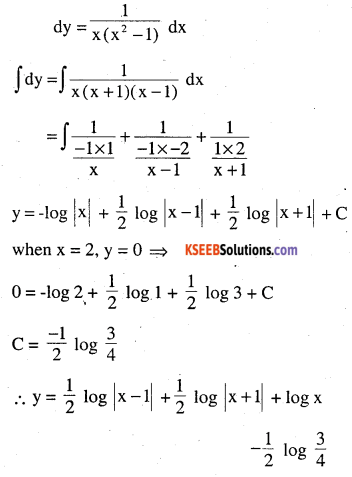Question 13.
$$\cos \left(\frac{\mathrm{dy}}{\mathrm{dx}}\right)=\mathrm{a}(\mathrm{a} \in \mathrm{R}) ; \mathrm{y}=\mathbf{2} \text { when } \mathrm{x}=0$$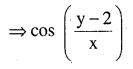Question 14.
$$\frac{d y}{d x}=y \tan x ; y=1 \text { when } x=0$$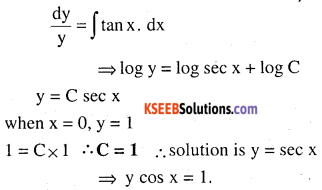Question 15.
Find the equation of a curve passing through the point (0,0) and whose differential equation is y’ – ex sin x.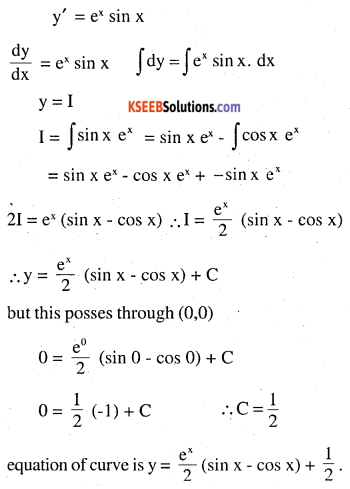Question 16.
For the differential equation $$\text { xy } \frac{d y}{d x}=(x+2)(y+2)$$,find the equation of curve passing through the point (1, -1).Question 17.
Find the equation of a curve passing through the point (0, -2), given that at any point
(x, y) on the curve, the product of the slope of its tangent and y coordinate of the point is equal to the x coordinate of the point.Question 18.
At any point (x, y) of a curve, the slope of the tangent is twice the slope of the line segment joining the point of contact to the point (- 4, -3). Find the equation of the curve given that it passes through (-2, 1).y + 3 = (x + 4)2 C
when x = -2, y = 1, 4 = (2)2 C
⇒ C = 1 Required equation is y + 3 = (x + 4)2

Question 19.
The volume of spherical balloon being inflated changes at a constant rate. If initially its radius is 3 units and after 3 seconds it is 6 units. Find the radius of balloon after t seconds.Question 20.
In a bank, principal increases continuously at the rate of r% per year. Find the value of r if ₹ 100 double itself in 10 years (log 2 = 0.6931).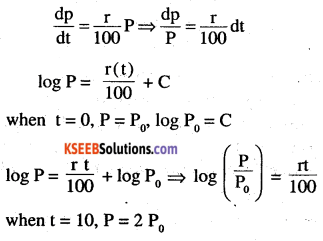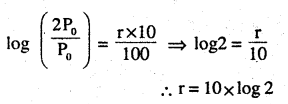∴ r=10x .6931=6.931
∴ The value of r if p = 100 doubles
2 P0 = 200 itself in 10 year is 6.931%.

Question 21.
In a bank, principal increases continuously at the rate of 5% per yean An amount of Rs 1000 is deposited with this bank, how much will it worth after 10 years (e0.5 = 1.648).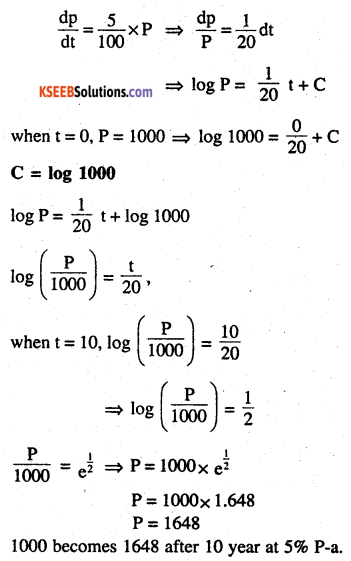Question 22.
In a culture, the bacteria count is 1,00,000. The number is increased by 10% in 2 hours. In how many hours will the count reach 2,00,000, if the rate of growth of bacteria is proportional to the number present?
Let P be the count of bacteria at the end of t hrsQuestion 23.
The general solutions of the differential equation $$\frac{d y}{d x}=e^{x+y} \text { is }$$
(A) $$\mathbf{e}^{x}+\mathbf{e}^{-y}=\mathbf{C}$$
(B) $$\mathbf{e}^{x}+\mathbf{e}^{y}=\mathbf{C}$$
(C) $$\mathbf{e}^{-x}+\mathbf{e}^{y}=\mathbf{C}$$
(D) $$\mathbf{e}^{x}+\mathbf{e}^{-y}=\mathbf{C}$$